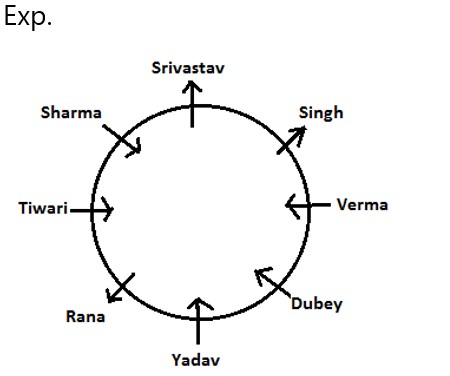# RRB PO Prelims Reasoning Ability Quiz – 25

## RRB PO Prelims Reasoning Ability Quiz

Reasoning Ability is a part of almost all major competitive exams in the country and is perhaps the most scoring section also. Aspirants who regularly practice questions have a good chance of scoring well in the Reasoning Ability Section. So here we are providing you with the RRB PO Reasoning Ability Quiz to help you prepare better. This RRB PO Reasoning Ability Quiz includes all of the most recent pattern-based questions, as well as Previous Year Questions. This RRB PO Reasoning Ability Quiz is available to you at no cost. Candidates will be provided with a detailed explanation of each question in this RRB PO Reasoning Ability Quiz. Candidates must practice this RRB PO Reasoning Ability Quiz to achieve a good score in the Reasoning Ability Section.

Directions (1-5): Study the given information and answer the following questions:

Eight Politicians – Singh, Sharma, Tiwari, Dubey, Rana, Verma, Yadav and Srivastav are sitting in a circle in a meeting at equal distances. Three Politicians on the circle are facing opposite side and other five are sitting facing the center of the circle. Sharma is sitting to the third right of Verma. Dubey is not near to Srivastav. Tiwari is sitting to the third left of Dubey, and Dubey sits second right of Singh and among these three Politicians one is facing opposite to the center of the circle. Two Politicians are sitting between Srivastav and Rana and two are sitting between Verma and Rana. Tiwari is sitting to the second left of Yadav, who is facing the center of the circle. Rana is not opposite to Verma and Tiwari.

1. Which of the following Politicians is facing to the opposite of the center?

(a)     Verma

(b)     Dubey

(c)     Rana

(d)     Sharma

(e)     None of these

Ans. c1. Who is sitting third to the left of Rana?

(a)     Dubey

(b)     Verma

(c)     Sharma

(d)     Srivastav

(e)     None of these

Ans. b1. If all the people change their positions with the person sitting opposite to them then who is now sitting second to the left of Dubey?

(a)     Rana

(b)     Singh

(c)     Verma

(d)     Srivastav

(e)     None of these

Ans. a1. Who is second right to Yadav?

(a)     Verma

(b)     Srivastav

(c)     Sharma or Verma

(d)     Dubey or Sharma

(e)     Srivastav or Dubey

Ans. a1. How many people are sitting b/w Srivastav and Dubey, counting in anticlockwise direction from Dubey?

(a)     Three

(b)     None

(c)     One

(d)     Two

(e)     None of these

Ans. dDirections (6-10): In these questions, relationship between different elements is. shown in the statements. The statements are followed by conclusions. Study the conclusions based on the given statements and select the appropriate answer.

1. Statement: P < L A > M = K E

Conclusions : I. K L   II. P < E

(a) Only conclusion II is true

(b) Either conclusion I or II is true

(c) Both conclusions I and II are true

(d) Neither conclusion I nor II is true

(e) Only conclusion I is true

Ans. d

Exp.

1. K=M<A≥L(False) II.  P<L≤A>M=K≥E(False)
1. Statements : P > R = A < Y; D< A

Conclusions : I. P > D II. D < Y

(a) Both conclusions I and II are true

(b) Only conclusion I is true

(c) Neither conclusion I nor II is true

(d) Either conclusion I or II is true

(e) Only conclusion II is true

Ans. a

Exp.

1. P>R=A>D(True) II.  D<A<Y(True)
1. Statements : P>R= A<Y; D<A

Conclusions : I. P < Y II. R D

(a) Both conclusions I and II are true

(b) Neither conclusion I nor II is true

(c) Only conclusion I is true

(d) Only conclusion II is true

(e) Either conclusion I or II is true

Ans. b

Exp.

1. P>R=A<Y(False) II.  R=A>D(False)
1. Statements : C R>A=S H; R< P< Q

Conclusions : I. C > S II. P < C

(a) Either conclusion I or II is true

(b) Both conclusions I and II are true

(c) Only conclusion II is true

(d) Neither conclusion I nor II is true

(e) Only conclusion I is true

Ans. e

Exp.

1. C≥R>A=S(True) II.  P>R≤C(False)

1. Statements : C R >A=SH; R<P<Q

Conclusions : I. H R II.. R < Q

(a) Both conclusions I and II are true

(b) Only conclusion II is true

(c) Only conclusion I is true

(d) Either conclusion I or II is true

(e) Neither conclusion I nor II is true

Ans. b

Exp.

1. H≥S=A<R(False) II. R<P<Q(True)

###Click to Buy Bank MahaCombo PackageRecommended PDF’s for:

#### Most important PDF’s for Bank, SSC, Railway and Other Government Exam : Download PDF Now

AATMA-NIRBHAR Series- Static GK/Awareness Practice Ebook PDF Get PDF here
The Banking Awareness 500 MCQs E-book| Bilingual (Hindi + English) Get PDF here
AATMA-NIRBHAR Series- Banking Awareness Practice Ebook PDF Get PDF here
Computer Awareness Capsule 2.O Get PDF here
AATMA-NIRBHAR Series Quantitative Aptitude Topic-Wise PDF Get PDF here
AATMA-NIRBHAR Series Reasoning Topic-Wise PDF Get PDF Here
Memory Based Puzzle E-book | 2016-19 Exams Covered Get PDF here
Caselet Data Interpretation 200 Questions Get PDF here
Puzzle & Seating Arrangement E-Book for BANK PO MAINS (Vol-1) Get PDF here
ARITHMETIC DATA INTERPRETATION 2.O E-book Get PDF here

3